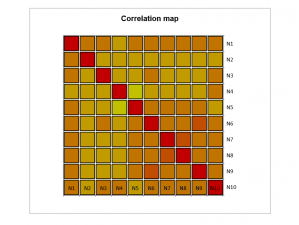# Zuverlässigkeitsanalyse

This features allows you to compute reliability indexes including Cronbach's Alpha. Perform reliability analysis in Excel using the XLSTAT add-on statistical software.## What is reliability analysis?

Reliability analysis is used in several areas, noticeably in social science. The terminology finds its origin in psychometry. We thus define a test made up of questions. Questions are called elements. Those elements are gathered within homogeneous constructs also called factors, measurement scales, latent variables or concepts. As an illustration, graphical skill might be a factor on which we wish to measure a level on a scale of measurement. The goal of the reliability analysis is to assess the reliability of this scale of measurement, or, in other words, that the construct questions are coherent and measure the same thing. In the case of the graphical skill, a question on mental calculus would downgrade the coherence of the scale.

To the statistician, questions are variables often measured on Likert-type scale (rating answers). Results of a test collected on a group of individuals are gathered in an individuals/variables array. To ensure compatibility with other areas such as quality control where the reliability analysis might also be used, those arrays are labelled observations/variables within XLSTAT.

Methods implemented in XLSTAT are used to estimate the internal consistency of a scale by making sure that results to different questions addressing the same phenomenon are coherent. Moreover, they also measure the reliability between two tests administered to the same individuals at two different times.

The internal analysis, allows to determine which elements of your survey are correlated by providing index related to the internal consistency of the scale but also to identify unnecessary elements and therefore to exclude them.

The split-half reliability analysis measures the equivalence between two parts of a test (parallel forms reliability). This type of analysis is used for two similar sets of items measuring the same thing, using the same instrument and with the same people.

The inter-rater analysis measures reliability by comparing each subject's evaluation variability to the total variability involving all subjects. This analysis has been developed based on the assumption that evaluations may vary according to the rater.

## Internal reliability

### Cronbach’s alpha

Cronbach's alpha index measures internal consistency, which is, how closely related a set of items are. It is considered to be a measure of scale reliability.

This index is the mathematical equivalent of the average of all correlations between 2 equal portions of the scale.

Many methodologists recommend a minimum alpha coefficient between 0.65 and 0.8 (or higher in many cases); those which are less than 0.5 are usually unacceptable.

XLSTAT also provides the standardized Alpha coefficient which is equivalent to the reliability that would be obtained if all items values were standardized (centered-reduced variables) before computing Cronbach’s alpha.

### Guttman's reliability coefficients (lambda 1-6)

Guttman developed six reliability coefficients: L1 to L6. Four of them (L1, L3, L5 and L6) are used to estimate internal consistency and the last two (L2 and L4) are used for split-half reliabilities:

• L1: Intermediate coefficient used in computing the other lambdas.
• L2: Estimation of the inter-score correlation in the case of parallel measurements. It is more complex than the Cronbach alpha and better represents the true reliability of the test.
• L3: Equivalent to Cronbach's alpha.
• L4: Guttman split-half reliability (See the description below).
• L5: Recommended when a single item strongly covaries with other items whereas those items don’t covary between each other.
• L6: Recommended when inter-item correlations are low compared to the item squared multiple correlations (becomes a better estimator as the number of elements increase).

## Split-Half reliability

### Spearman-Brown reliability

Another way to calculate the reliability of a scale is to randomly split it into two parts. If the scale is perfectly reliable, we expect the two halves to be perfectly correlated (R = 1). Bad reliability leads to imperfect correlations. Split-Half reliability can be estimated using the Spearman-Brown coefficient. When the two halves have different sizes, a more accurate estimate of this coefficient is used (Horst’s Formula).

### Guttman split-half reliability (Split-half model):

Guttman's split-half reliability (L4) is similar to the Spearman-Brown coefficient, but does not consider the reliabilities or variances to be equal in both halves (Tau-equivalence).

## Inter-rater reliability

### Intra-class Correlation Coefficient (ICC)

Reliability can also be tested using the Inter-rater reliability, with coefficients such as the Intra-class Correlation Coefficient (ICC). This coefficient has several variants but can be generally defined as the part of variance of an observation due to the variability between subjects. ICC values typically vary between 0 and 1.

ICC values are high when there is little variation between the scores given for each question by raters.### analysieren sie ihre daten mit xlstat

kostenlose 14-tage-testversion

Enthalten ind

Verwandte Funktionen